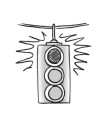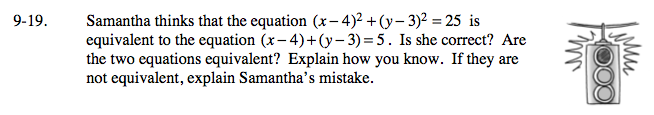### Home > A2C > Chapter 9 > Lesson 9.1.1 > Problem9-19

9-19.

Samantha thinks that the equation (x − 4)2 + (y − 3)2 = 25 is equivalent to the equation (x − 4) + (y − 3) = 5. Is she correct? Are the two equations equivalent? Explain how you know. If they are not equivalent, explain Samantha's mistake. Homework Help ✎Try substituting (0, 0) into both equations.